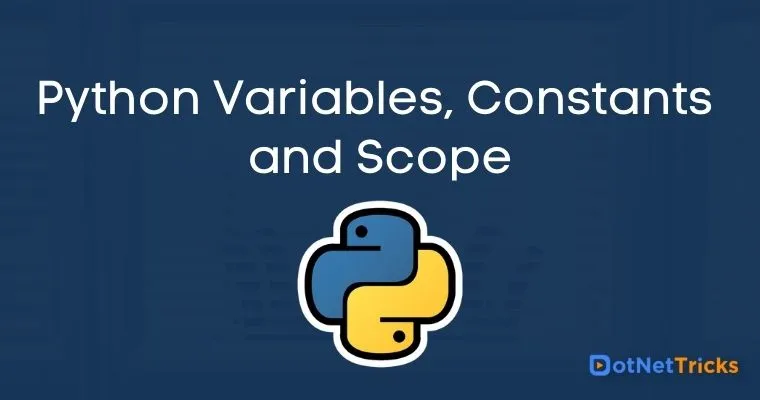# Python Variables, Constants and Scope

02 Sep 2022
Beginner
12.2K Views

Variable is a concept we have read in all the programming languages it's not different for Python. Variable is just a way to label a memory location in the computer. Each variable is associated with an identified which must be unique. We can think variable as a container that holds data which can be manipulated later as per the need of the program. In layman term, we can say a bookshelf where we can keep any books and can change them anytime as per our needs. Python works on value by reference simply it assigns the reference of the object to a variable.

## Declaration

There isn’t any concept of declaring or initializing a variable in Python. It happens automatically when you assign a value to a variable.

### Assigning a value

Assignment operator (=) is used to assign a value to a variable

Example 1 : Assigning value to variable in Python

``` url = "dotnettricks.com"

print(url)
```
Output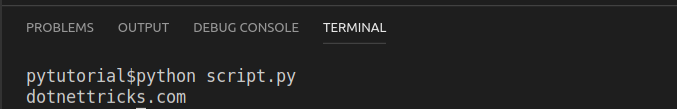Example 2 : Manipulating value of variable

``` url = "dotnettricks.com"

url = "tricks.com"
print(url)
```
Output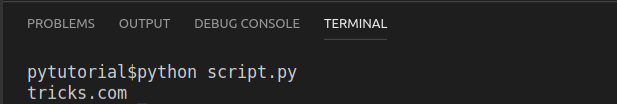Here we assigning a value to a variable and then changed that value.

Example 3 : Multiple values assignment in single statement.Python has a very simpler syntax for multiple assignment

```
a = b = c = 1, True, "Hello"

print(a)
print(b)
print(c)
```
Output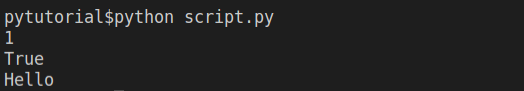## Constants

Constants are another type of variable whose value can’t be changed once assigned.It will through error if you try to assign the value again.

### Assigning value to a constant

Constants are declared and assigned on a module level in Python. Module can contain constants, functions etc. later they are imported inside the main file. Constants are written in capital letters and separated by underscore for words.

Example 3 : Constants in Python

Create constant.py
``` PI = 3.14
GRAVITY = 9.8
```
Create main.py
``` import constant

print(constant.PI)
print(constant.GRAVITY)
```
Output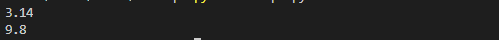In above program we first declared all the constants needed and assigned some value to them. After that, we create our main module in which we will import those constants and use. Importing can be done with import keyword. In reality we don’t use constants in python but we store sometimes configurations like this that can be used throughout the project.

## Rules and Naming conventions – Variable and Constants

1. Use sensible names for variables that makes program more readable and understandable.

2. Use camelCase convention for variables. It starts with lowercase letter. For example:

```myBook
myName
```
3. As earlier said use Capital letters for Constants.

```
PI
GRAVITY
MASS
```
4. Avoid symbols and special characters like -!, #, \$, % etc.

5. Starting a variable name with digit is not acceptable.

6. Constants are put into different module file whose values are not meant to be changed.

7. Constants should have combination of lowercase or uppercase or digits or underscore. For example

```
small_case
UP_CASE
camelCase
TitleCase
```

## Scope of Variable

Scope of a variable in python is not different than other programming languages. Here also variable follows same rule i.e., if a variable is defined inside a function then its scope is inside the function only and variables which are declared outside of any function block globally do possess a global scope.

Example 1 : Global Scope
```
value1 = 20 #Globally Declared

def ChangeValue():
print(value1) #Will use Global Value

ChangeValue() #Calling a function
print(value1) #Printing its value again
```
Output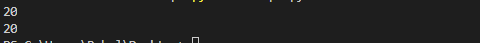In above program we declared a variable globally and then we wrote a function inside that we printed the value of variable. After the function we again printed the value of variable. The variable was declared globally so we can use it inside function.

Example 2 : Local Scope
```
value1 = 20 #Globally Declared

def ChangeValue():
value1 = 30 # Local scope declaration
print(value1) #Will use localScope

ChangeValue() #Calling a function
print(value1) #Printing its value again
```
Output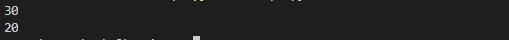In above program we declared variable with same name inside a function. If you remember how we manipulated the value of variable but here it didn’t work because Python considered it as declaration inside function scope i.e., local scope.

Note :- Explanation about function is not in scope of this article we will discuss it later

##### Summary

In this article we learned about variables and constants in Python and what are different rules and naming convention we should follow while declaring. Naming conventions are more or less similar to other languages the major advantage or we can say ease of declaration is we don’t need to mention datatype while declaration we can just assign value and Python will take care of the datatype.

Learn to Crack Your Technical Interview